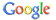web uni-protokolle.de powered by# Asymptotic Modelling of Fluid Flow Phenomena (Fluid Mechanics and Its Applications, Volume 64)von R. Kh ZeytounianKategorie: AllgemeinISBN: 140200432X Kommentar abgeben

Synopsis Devoted entirely to asymptotic modelling of fluid flow phenomena, this book deals with the art of the asymptotic modelling of Newtonian laminar fluid flows. This asymptotic modelling consists of deriving fluid flow model problems in such a way that they become amenable to mathematical analysis and to numerical simulations. The main goal of the text is modelling and not the presentation of solutions. One may assume that for some time to come the further expansion of the capabilities of numerical simulations will depend on, or will at least be related to, the development of asymptotic modelling. This book includes the basic aspects, recent developments and the current issues important to the asymptotic modelling of fluid flow phenomena.

 Allgemein > Asymptotic Modelling of Fluid Flow Phenomena (Fluid Mechanics and Its Applications, Volume 64)

HTML-Code zum Verweis auf diese Seite:
<a href="http://www.uni-protokolle.de/buecher/isbn/140200432X/">Asymptotic Modelling of Fluid Flow Phenomena (Fluid Mechanics and Its Applications, Volume 64) </a>